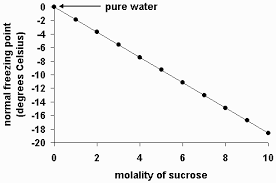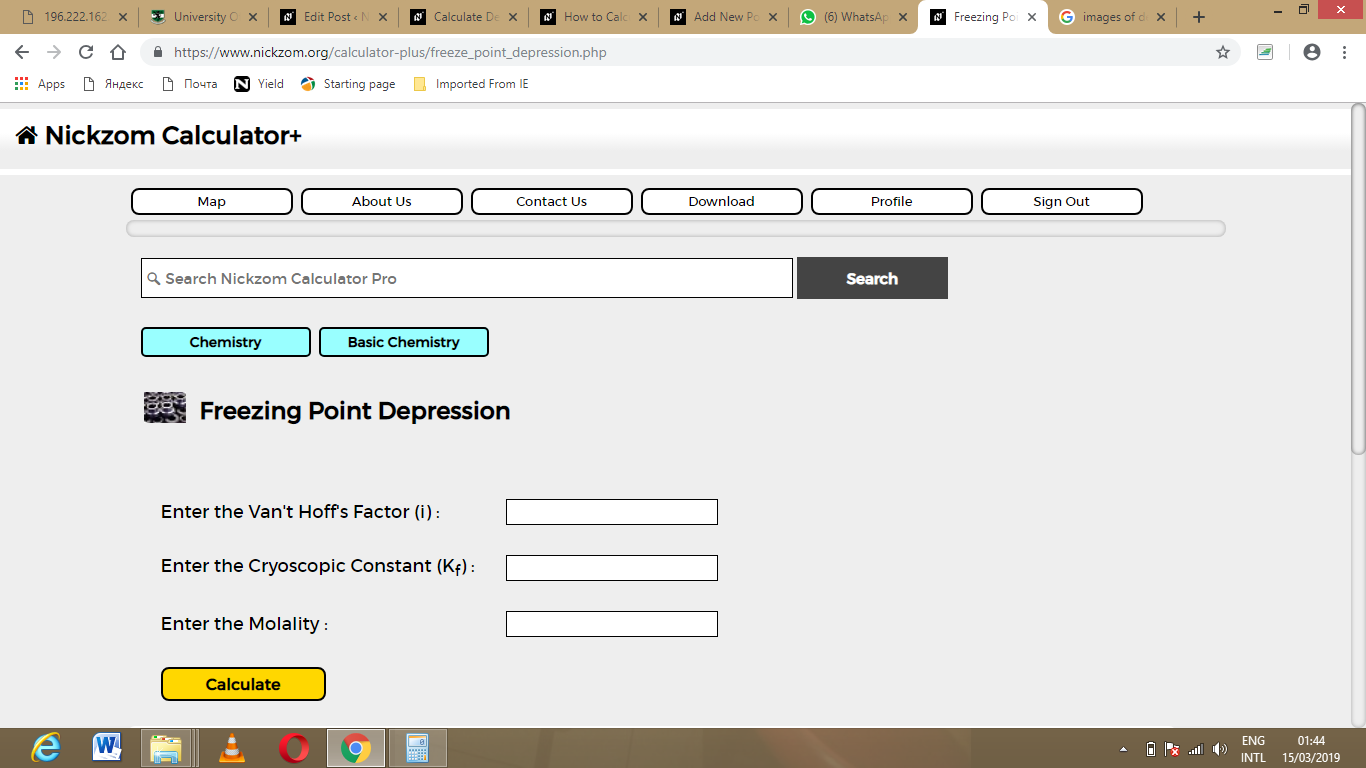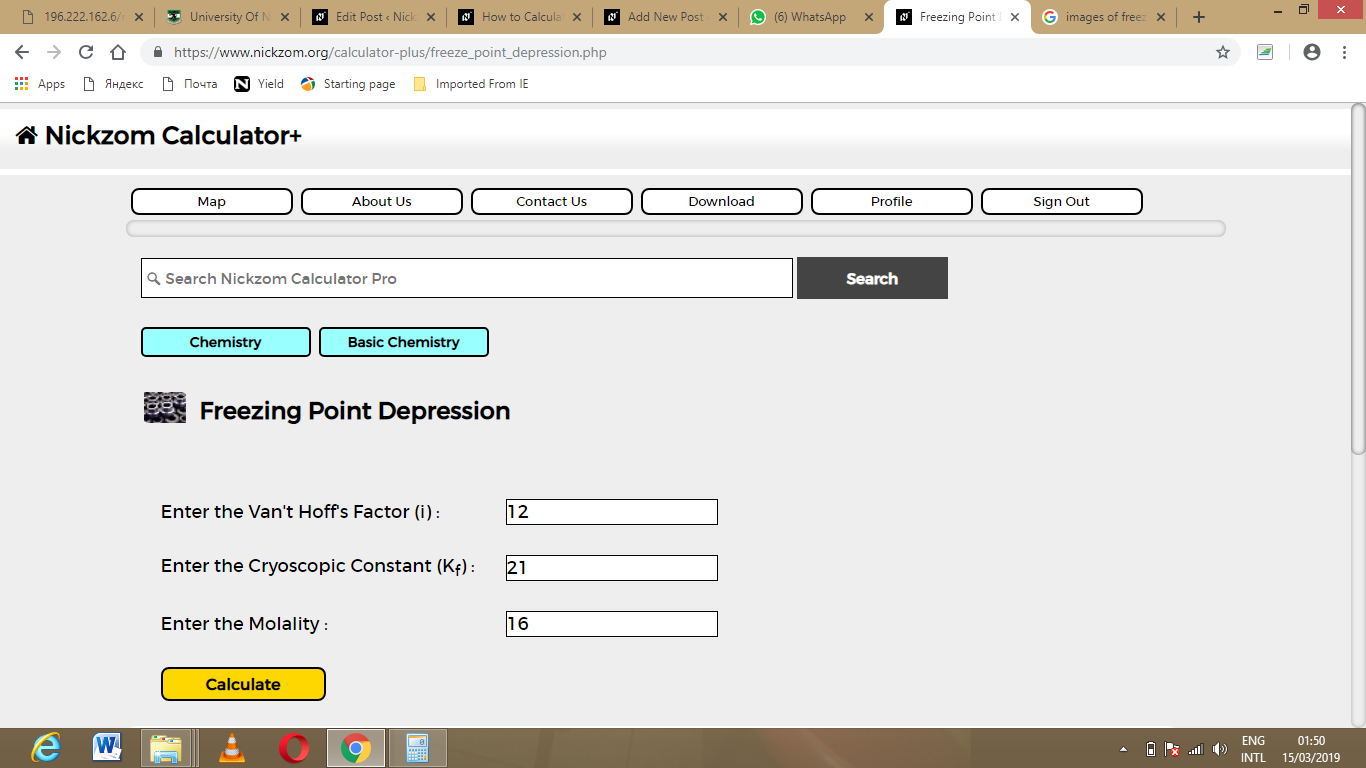# How to Calculate and Solve for Van’t Hoff’s Factor, Cryoscopic Constant, Molality and Freezing Point Depression | Nickzom CalculatorThe image above represents the freezing point depression.

To compute for the freezing point depression, three essential parameters are needed and these parameters are Van’t Hoff’s Factor (i), cryoscopic constant (Kf) and molality.

The formula for calculating freezing point depression:

δTf = iKf x Molality

Where;
δTf = Freezing point depression
i = Van’t Hoff’s Factor
Kf = cryoscopic constant
Molality

Let’s solve an example;
Find the freezing point depression when the van’t hoff’s factor is 12, cryoscopic constant is 21 with a molality of 16.

δTf = iKf x Molality
δTf = (12 x 21) x 16
δTf = 252 x 16
δTf = 4032

Therefore, the freezing point depression is 4032 °C m-1.

Nickzom Calculator – The Calculator Encyclopedia is capable of calculating the freezing point depression.

To get the answer and workings of the freezing point depression using the Nickzom Calculator – The Calculator Encyclopedia. First, you need to obtain the app.

You can get this app via any of these means:

To get access to the professional version via web, you need to register and subscribe for NGN 1,500 per annum to have utter access to all functionalities.
You can also try the demo version via https://www.nickzom.org/calculator

Once, you have obtained the calculator encyclopedia app, proceed to the Calculator Map, then click on Basic Chemistry under ChemistryNow, Click on Freezing Point Depression under Basic ChemistryThe screenshot below displays the page or activity to enter your values, to get the answer for the freezing point depression according to the respective parameters which are the Van’t Hoff’s Factor (i), Cryoscopic Constant (Kf) and Molality.Now, enter the values appropriately and accordingly for the parameters as required by the example above where the Van’t Hoff’s Factor (i) is 12, Cryoscopic Constant (Kf) is 21 and Molality is 16.Finally, Click on CalculateAs you can see from the screenshot above, Nickzom Calculator – The Calculator Encyclopedia solves for the freezing point depression and presents the formula, workings and steps too.